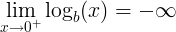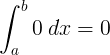# Zero Number (0)

### Zero number definition

Zero is a number used in mathematics to describe no quantity or null quantity.

When there are 2 apples on the table and we take the 2 apples, we can say that there are zero apples on the table.

The zero number is not positive number and not negative number.

The zero is also a placeholder digit in other numbers (e.g: 40,103, 170).

#### Is zero a number?

Zero is a number. It is not positive nor negative number.

#### Zero digit

The zero digit is used as a placeholder when writing numbers.

For example:

204 = 2×100+0×10+4×1

### Zero number history

#### Who invented the zero number?

The modern 0 symbol was invented in India in the 6-th century, used later by the Persians and Arabs and later in Europe.

#### Symbol of zero

The zero number is denoted with the 0 symbol.

The Arabic numeral system uses the ٠ symbol.

### Zero number properties

x represents any number.

Operation Rule Example

x + 0 = x

3 + 0 = 3

x - 0 = x

3 - 0 = 3

x × 0 = 0

5 × 0 = 0

##### Division

0 ÷ x = 0 , when x ≠ 0

0 ÷ 5 = 0

x ÷ 0  is undefined

5 ÷ 0 is undefined

0 x = 0

05 = 0

x 0 = 1

50 = 1

0 = 0

##### Logarithm

logb(0) is undefined0! = 1

sin 0º = 0

cos 0º = 1

tan 0º = 0

0' = 0

##### Integral

∫ 0 dx = 0 + CAddition of a number plus zero is equal to the number:

x + 0 = x

For example:

5 + 0 = 5

#### Zero subtraction

Subtraction of a number minus zero is equal to the number:

x - 0 = x

For example:

5 - 0 = 5

#### Multiplication by zero

Multiplication of a number times zero is equal to zero:

x × 0 = 0

For example:

5 × 0 = 0

#### Number divided by zero

Division of a number by zero is not defined:

x ÷ 0 is undefined

For example:

5 ÷ 0 is undefined

#### Zero divided by a number

Division of a zero by a number is zero:

0 ÷ x = 0

For example:

0 ÷ 5 = 0

#### Number to the zero power

The power of a number raised by zero is one:

x0 = 1

For example:

50 = 1

#### Logarithm of zero

The base b logarithm of zero is undefined:

logb(0) is undefined

There is no number we can raise the base b with to get zero.

Only the limit of the base b logarithm of x, when x converges zero is minus infinity:### Sets that contain zero

Zero is an element of the natural numbers, integer numbers, real numbers and complex numbers sets:

Set Set membership notation
Natural numbers (non negative) 0 ∈ ℕ0
Integer numbers 0 ∈ ℤ
Real numbers 0 ∈ ℝ
Complex numbers 0 ∈ ℂ
Rational numbers 0 ∈ ℚ

#### Is zero even or odd number?

The set of even numbers is:

{... ,-10, -8, -6, -4, -2, 0, 2, 4, 6, 8, 10, ...}

The set of odd numbers is:

{... ,-9, -7, -5, -3, -1, 1, 3, 5, 7, 9, ...}

Zero is an integer multiple of 2:

0 × 2 = 0

Zero is a member of the even numbers set:

0 ∈ {2k, k∈ℤ}

So zero is an even number and not an odd number.

#### Is zero a natural number?

There are two definitions for the natural numbers set.

The set of non negative integers:

0 = {0,1,2,3,4,5,6,7,8,...}

The set of positive integers:

1 = {1,2,3,4,5,6,7,8,...}

Zero is a member of the set of non negative integers:

0 ∈ ℕ0

Zero is not a member of the set of positive integers:

0 ∉ ℕ1

#### Is zero a whole number?

There are three definitions for the whole numbers:

The set of integer numbers:

ℤ = {0,1,2,3,4,5,6,7,8,...}

The set of non negative integers:

0 = {0,1,2,3,4,5,6,7,8,...}

The set of positive integers:

1 = {1,2,3,4,5,6,7,8,...}

Zero is a member of the set of integer numbers and the set of non negative integers:

0 ∈ ℤ

0 ∈ ℕ0

Zero is not a member of the set of positive integers:

0 ∉ ℕ1

#### Is zero an integer number?

The set of integer numbers:

ℤ = {0,1,2,3,4,5,6,7,8,...}

Zero is a member of the set of integer numbers:

0 ∈ ℤ

So zero is an integer number.

#### Is zero a rational number?

A rational number is a number that can be expressed as the quotient of two integer numbers:

ℚ = {n/m; n,m∈ℤ}

Zero can be written as a quotient of two integer numbers.

For example:

0 = 0/3

So zero is a rational number.

#### Is zero a positive number?

A positive number is defined as a number that is greater than zero:

x > 0

For example:

5 > 0

Since zero is not greater than zero, it is not a positive number.

#### Is zero a prime number?

The number 0 is not a prime number.

Zero is not a positive number and has infinite number of divisors.

The lowest prime number is 2.